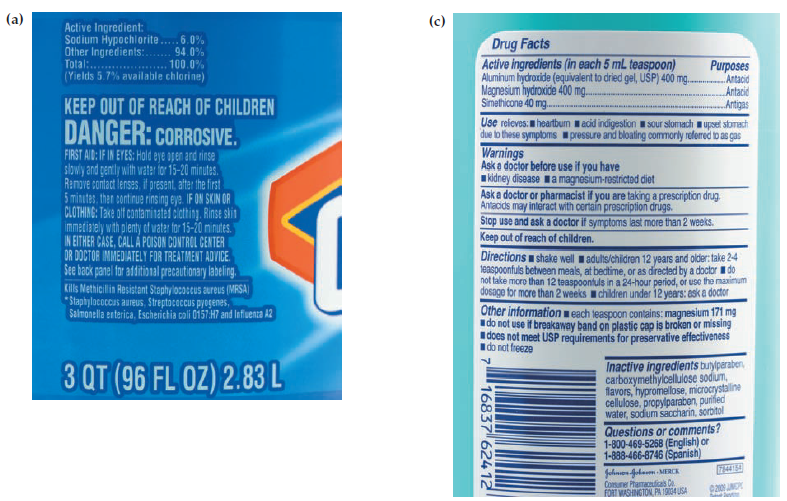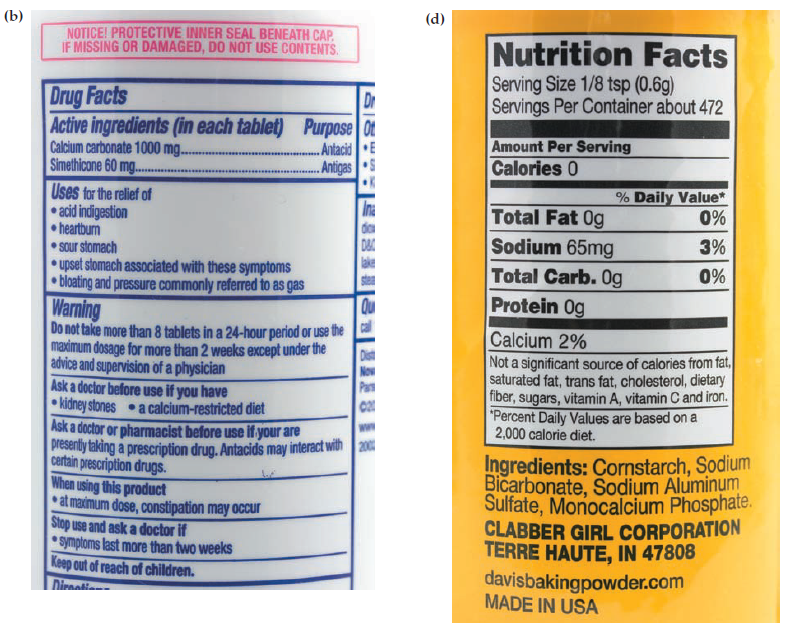×
Get Full Access to Introductory Chemistry - 5 Edition - Chapter 5 - Problem 107p
Get Full Access to Introductory Chemistry - 5 Edition - Chapter 5 - Problem 107p

×

# Examine each consumer product label. Write chemicalISBN: 9780321910295 34

## Solution for problem 107P Chapter 5

Introductory Chemistry | 5th Edition

• Textbook Solutions
• 2901 Step-by-step solutions solved by professors and subject experts
• Get 24/7 help from StudySoup virtual teaching assistantsIntroductory Chemistry | 5th Edition

4 5 1 387 Reviews
30
5
Problem 107P

Examine each consumer product label. Write chemical formulas for as many of the compounds as possible based on what you have learned in this chapter.Step-by-Step Solution:
Step 1 of 3

Solution 107P

Here, we are going to write the chemical formulas of compounds in each consumer product label.

The list of chemical compounds along with their molecular formulas identified in the given consumer labels are:

1. Sodium hypochlorite - NaClO
2. Calcium carbonate - CaCO3
3. Aluminium hydroxide - Al(OH)3
4. Magnesium hydroxide - Mg(OH)2
5. Sodium bicarbonate - NaHCO3
6. Sodium aluminium sulfate - NaAl(SO4)2.12H2O
7. Monocalcium phosphate - Ca(H2PO4)2
8. Simethicone - C6H18O3Si3
9. Cornstarch - C27H48O20

-----------------

Step 2 of 3

Step 3 of 3

##### ISBN: 9780321910295

Introductory Chemistry was written by and is associated to the ISBN: 9780321910295. The answer to “?Examine each consumer product label. Write chemical formulas for as many of the compounds as possible based on what you have learned in this chapter.” is broken down into a number of easy to follow steps, and 25 words. This full solution covers the following key subjects: based, Chapter, chemical, compounds, Consumer. This expansive textbook survival guide covers 19 chapters, and 2046 solutions. Since the solution to 107P from 5 chapter was answered, more than 359 students have viewed the full step-by-step answer. This textbook survival guide was created for the textbook: Introductory Chemistry, edition: 5. The full step-by-step solution to problem: 107P from chapter: 5 was answered by , our top Chemistry solution expert on 05/06/17, 06:45PM.

Unlock Textbook Solution